Transforming Numerical Methods Education for the STEM Undergraduate MOOC | MOBILE | VIDEOS | BLOG | YOUTUBE | TWITTER | COMMENTS | ANALYTICS | ABOUT | CONTACT | COURSE WEBSITES | BOOKS | MATH FOR COLLEGE
 MULTIPLE CHOICE TEST LU DECOMPOSITION SIMULTANEOUS LINEAR EQNS

Q1. The LU decomposition method is computationally more efficient than Na�ve Gauss elimination method for solving

a single set of simultaneous linear equations

multiple sets of simultaneous linear equations with different coefficient matrices and the same right hand side vectors.

multiple sets of simultaneous linear equations with the same coefficient matrix but different right hand sides.

less than ten simultaneous linear equations.

Q2. The lower triangular matrix [L] in the [L][U] decomposition of the matrix given belowisQ3. The upper triangular matrix [U] in the [L][U] decomposition of the matrix given belowisQ4. For a given 2000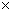2000 matrix [A], assume that it takes about 15 seconds to find the inverse of [A] by use of the [L][U] decomposition method, that is, finding the [L][U] once, and then doing forward substitution and back substitution 2000 times using the 2000 columns of the identity matrix as the right hand side vector.  The approximate time, in seconds, that it will take to find the inverse if found by repeated use of the Naive Gauss elimination method, that is, doing forward elimination and back substitution 2000 times by using the 2000 columns of the identity matrix as the right hand side vector is most nearly

300

1500

7500

30000

Q5. The algorithm for solving the set of n equations [A][X] = [C], where [A] = [L][U] involves solving

[L][Z] = [C] by forward substitution.  The algorithm to solve [L][Z]=[C] is given by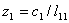for i from 2 to n do

sum = 0

for j from 1 to i do

sum = sum +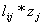end do

zi = (ci � sum) / lii

end dofor i from 2 to n do

sum = 0

for j from 1 to (i-1) do

sum = sum +end do

zi = (ci � sum) / lii

end dofor i from 2 to n do

for j from 1 to (i-1) do

sum = sum +end do

zi = (ci � sum) / lii

end do

for i from 2 to n do

sum = 0

for j from 1 to (i-1) do

sum = sum +end do

zi = (ci � sum) / lii

end do

Q6. To solve boundary value problems, the finite difference methods are used resulting in simultaneous linear equations with tridiagonal coefficient matrices.  These are solved using the specialized [L][U] decomposition method.  The set of equations in matrix form with a tridiagonal coefficient matrix for,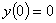,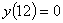,

using the finite difference method with a second order accurate central divided difference method and a step size of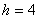isMultiple choice questions on other topics

 AUDIENCE |  AWARDS  |  PEOPLE  |  TRACKS  |  DISSEMINATION  |  PUBLICATIONS Copyrights: University of South Florida, 4202 E Fowler Ave, Tampa, FL 33620-5350. All Rights Reserved. Questions, suggestions or comments, contact kaw@eng.usf.edu  This material is based upon work supported by the National Science Foundation under Grant#0126793, 0341468, 0717624,  0836981, , 0836805, 1322586.  Any opinions, findings, and conclusions or recommendations expressed in this material are those of the author(s) and do not necessarily reflect the views of the National Science Foundation.  Other sponsors include Maple, MathCAD, USF, FAMU and MSOE.  Based on a work at http://mathforcollege.com/nm.  Holistic Numerical Methods licensed under a Creative Commons Attribution-NonCommercial-NoDerivs 3.0 Unported License. ANALYTICS